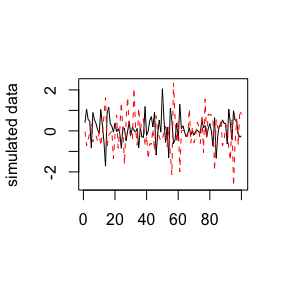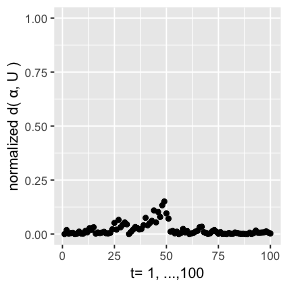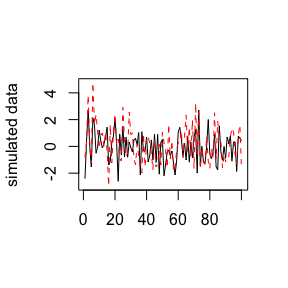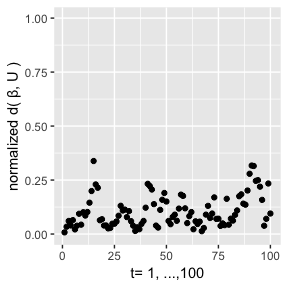# SMFilter version 1.0.3 (Red Filter)

The package implements the filtering algorithms for the state-space models on the Stiefel manifold. It also implements sampling algorithms for uniform, vector Langevin-Bingham and matrix Langevin-Bingham distributions on the Stiefel manifold. You can also find the package on CRAN, see

SMFilter@CRAN

and the corresponding paper

State-Space Models on the Stiefel Manifold with a New Approach to Nonlinear Filtering

## How to install

You can either install the stable version from CRAN

install.packages("SMFilter")

or install the development version from GitHub

devtools::install_github("yukai-yang/SMFilter")

provided that the package “devtools” has been installed beforehand.

## Example

After installing the package, you need to load (attach better say) it by running the code

library(SMFilter)

You can first check the information and the current version number by running

version()
#> SMFilter version 1.0.3 (Red Filter)

Then you can take a look at all the available functions and data in the package

ls( grep("SMFilter", search()) )
#>  "FDist2"       "FilterModel1" "FilterModel2" "SimModel1"
#>  "SimModel2"    "rmLB_sm"      "runif_sm"     "rvlb_sm"
#>  "version"

### Type one model

For details, see

?SimModel1

First we can use the package to sample from the type one model. To this end, we shall initialize by running

set.seed(1) # control the seed
iT = 100 # sample size
ip = 2 # dimension of the dependent variable
ir = 1 # rank number
iqx = 3 # dimension of the independent variable x_t
iqz=0 # dimension of the independent variable z_t
ik = 0 # lag length
method='max_3' # the optimization methond to use, for details, see FilterModel1
Omega = diag(ip)*.1 # covariance of the errors
vD = 50 # diagonal of the D matrix

Then we initialize the data and some other parameters

if(iqx==0) mX=NULL else mX = matrix(rnorm(iT*iqx),iT, iqx)
if(iqz==0) mZ=NULL else mZ = matrix(rnorm(iT*iqz),iT, iqz)
if(ik==0) mY=NULL else mY = matrix(0, ik, ip)
alpha_0 = matrix(c(runif_sm(num=1,ip=ip,ir=ir)), ip, ir)
beta = matrix(c(runif_sm(num=1,ip=ip*ik+iqx,ir=ir)), ip*ik+iqx, ir)
if(ip*ik+iqz==0) mB=NULL else mB = matrix(c(runif_sm(num=1,ip=(ip*ik+iqz)*ip,ir=1)), ip, ip*ik+iqz)

Then we can simulate from the model

ret = SimModel1(iT=iT, mX=mX, mZ=mZ, mY=mY, alpha_0=alpha_0, beta=beta, mB=mB, vD=vD, Omega=Omega)

Have a look at the simulated data

matplot(ret$dData[,1:ip], type="l", ylab="simulated data")Then let’s apply the filtering algorithm on the data fil = FilterModel1(mY=as.matrix(ret$dData[,1:ip]), mX=mX, mZ=mZ, beta=beta, mB=mB, Omega=Omega, vD=vD, U0=alpha_0, method=method)

Then we compare the filtered modal orientations with the true ones in terms of the Frobenius distance.### Type two model

For details, see

?SimModel2

iT = 100
ip = 2
ir = 1
iqx = 4
iqz=0
ik = 0
Omega = diag(ip)*.1
vD = 50

Then we initialize the data and some other parameters

if(iqx==0) mX=NULL else mX = matrix(rnorm(iT*iqx),iT, iqx)
if(iqz==0) mZ=NULL else mZ = matrix(rnorm(iT*iqz),iT, iqz)
if(ik==0) mY=NULL else mY = matrix(0, ik, ip)
alpha = matrix(c(runif_sm(num=1,ip=ip,ir=ir)), ip, ir)
beta_0 = matrix(c(runif_sm(num=1,ip=ip*ik+iqx,ir=ir)), ip*ik+iqx, ir)
if(ip*ik+iqz==0) mB=NULL else mB = matrix(c(runif_sm(num=1,ip=(ip*ik+iqz)*ip,ir=1)), ip, ip*ik+iqz)

Then we can simulate from the model

ret = SimModel2(iT=iT, mX=mX, mZ=mZ, mY=mY, alpha=alpha, beta_0=beta_0, mB=mB, vD=vD)

And then have a look at the simulated data

matplot(ret$dData[,1:ip], type="l",ylab="simulated data")Apply the filtering algorithm on the data fil = FilterModel2(mY=as.matrix(ret$dData[,1:ip]), mX=mX, mZ=mZ, alpha=alpha, mB=mB, Omega=Omega, vD=vD, U0=beta_0, method=method)

Then we compare the filtered modal orientations with the true ones in terms of the Frobenius distance.